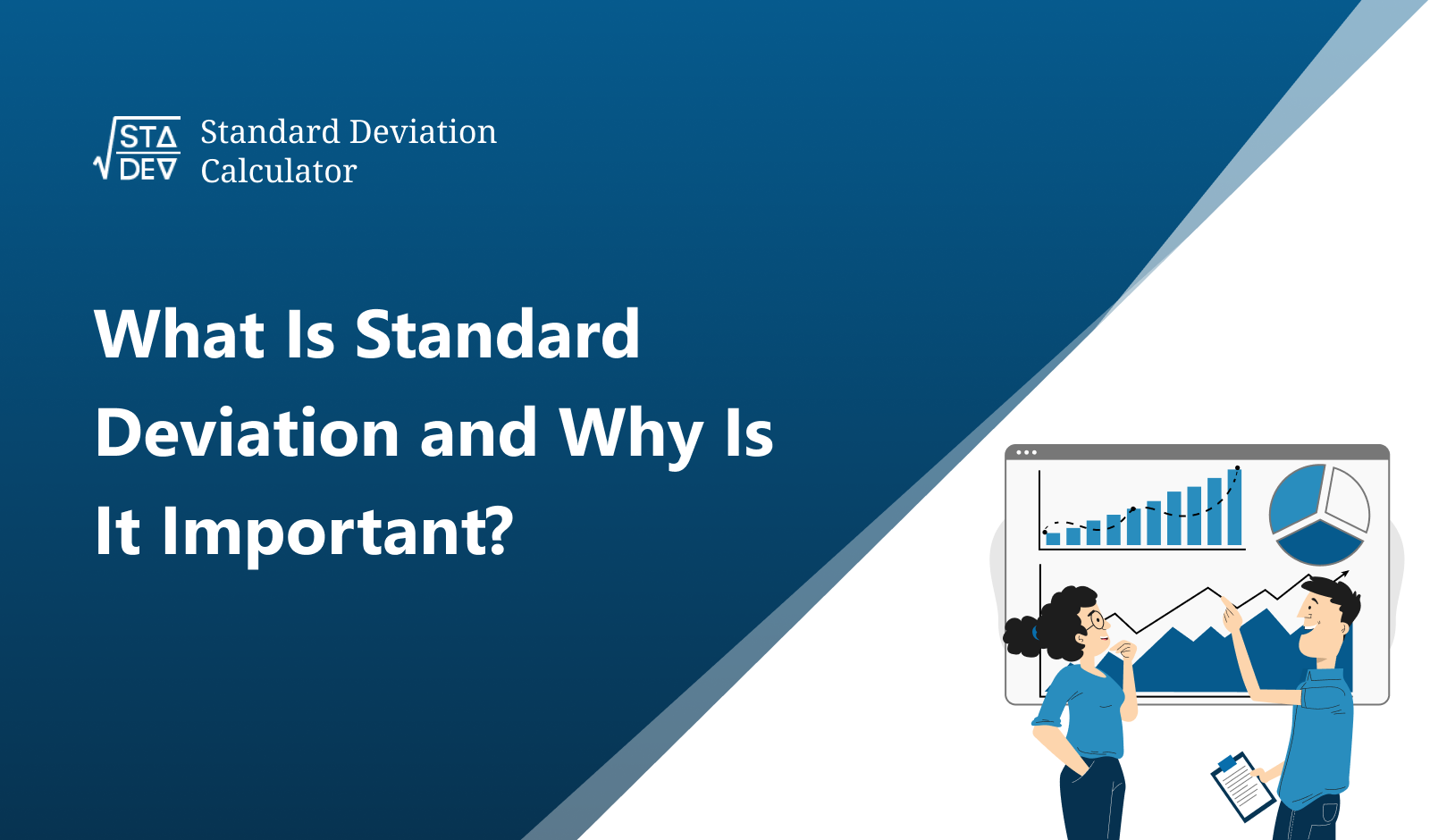Jun 1, 2023
What Is Standard Deviation and Why Is It Important?In the world of statistics and data analysis, understanding various terms and measures can be a daunting task. However, there are a few fundamental concepts that form the backbone of any statistical analysis - one of these is the Standard Deviation.

Today, we're going to break down this concept, understand its importance, and introduce you to a handy tool that can help with your calculations - the Standard Deviation Calculator.

## What Is Standard Deviation?

In simplest terms, the Standard Deviation is a statistical measure that reflects how much variation or dispersion exists in a set of values. It provides an insight into the degree to which individual data points in a dataset may diverge from the mean, or average.

For example:

Consider two data sets: Set 1 (5, 5, 5, 5, 5) and Set 2 (0, 5, 10, 15, 20). Both sets have the same mean of 5, but the values in Set 2 are spread out more. This dispersion is quantified by the Standard Deviation, which in this case is 0 for Set 1 (indicating no dispersion) and approximately 7.07 for Set 2 (indicating higher dispersion).

## Why Is Standard Deviation Important?

The importance of Standard Deviation lies in its diverse applications across numerous fields.

### Understanding Variability:

The most basic use of the Standard Deviation is to understand the variability in a dataset. If the individual observations vary greatly from the group mean, the Standard Deviation will be extensive, and vice versa. This helps us gauge the consistency within a dataset.

### Comparing Data:

Standard Deviation is instrumental in comparing different data sets. It provides a summary of the differences in their respective data distribution, indicating whether the mean is a representative depiction of the entire group.

### Identifying Outliers:

A critical application of Standard Deviation lies in identifying outliers in a dataset. Outliers, which significantly deviate from the mean, can distort the results of data analysis. Recognizing these outliers aids in refining our data analysis.

### Quality Assurance and Control:

In industries, Standard Deviation serves as a pillar of quality assurance and control. For instance, if a product's dimensions have a large Standard Deviation, it means the product sizes aren't consistent. This inconsistency could potentially affect the product’s functionality or customer satisfaction.

### Risk Assessment:

In financial scenarios, the Standard Deviation is a key market volatility indicator. It offers insights into the risk associated with various investment opportunities, thus serving as an important tool in predictive analysis.

## How To Calculate Standard Deviation?

Calculating Standard Deviation manually can be a time-consuming process, especially with larger data sets. But don't worry! Our Standard Deviation Calculator is here to make your life easier. This tool not only calculates the Standard Deviation but also provides other valuable statistical data.

## Wrapping Up:

The Standard Deviation is a powerful and versatile tool in data analysis. Understanding its concept and implications can significantly enhance the accuracy of your statistical analyses. Remember, our Standard Deviation Calculator is always there to assist you in your calculations.

Stay curious, and keep exploring!